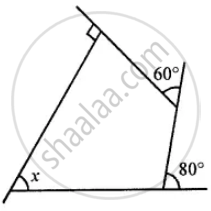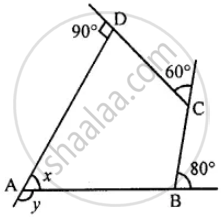# Use the Following Figure to Find the Value of X - Mathematics

Sum

Use the following figure to find the value of x#### Solution

The sum of exterior angles of a quadrilateral⇒ y + 80° + 60° + 90° = 360°

⇒ y + 230° = 360°

⇒ y = 360° – 230° = 130°

At vertex A,

∠y + ∠x = 180° (Linear pair)

x = 180° – 130°

⇒ x = 50°

Is there an error in this question or solution?

#### APPEARS IN

Selina Concise Mathematics Class 8 ICSE
Chapter 16 Understanding Shapes
Exercise 16 (C) | Q 13 | Page 188